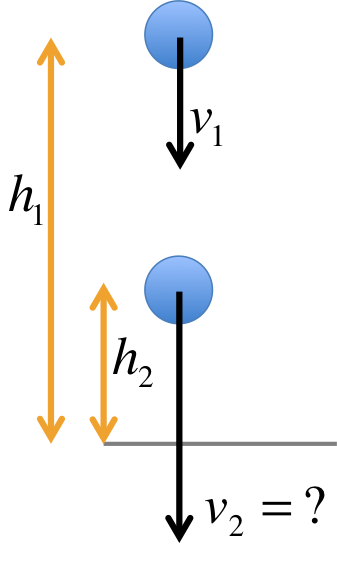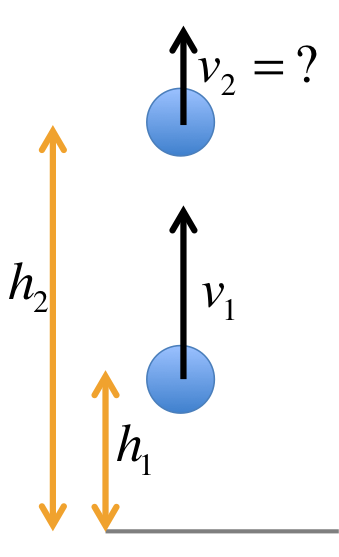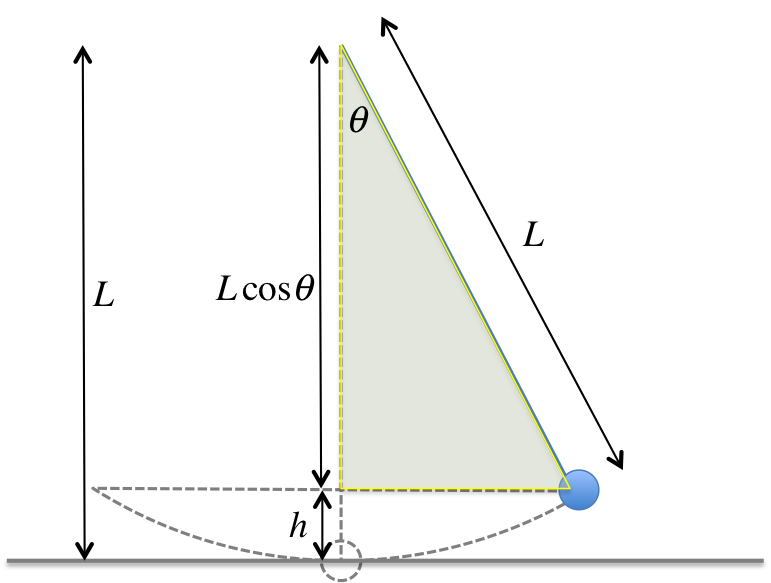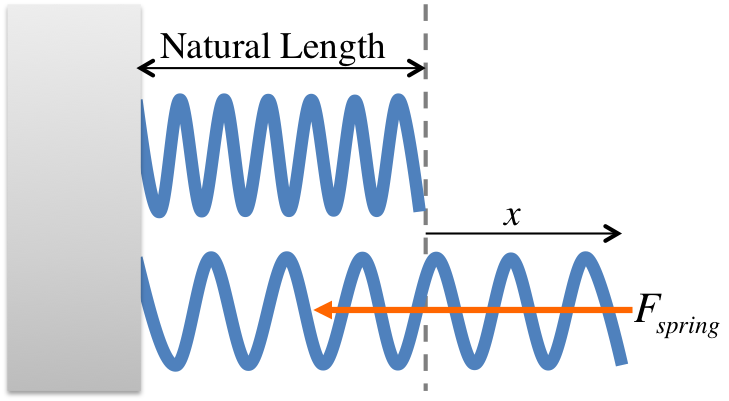# PHYS 170

Lecture 7 - Potential Energy

## Introduction

There are two types of mechanical energy, kinetic energy and potential energy. For now we will focus on gravitational potential energy: $$PE_g = mgh$$ The height $$h$$ is measure from an arbitrary reference level (say the ground). The higher an object is, the more gravitational potential energy it has. Together with kinetic energy $$KE=\frac{1}{2}mv^2$$ we can apply the law of conservation of energy to solve many problems. As with all other types of energy, its SI unit is $$J$$.

### Try It Yourself (click to show)

$$5m$$ above ground: $$PE = mgh = (10)(9.8)(5) = 490J$$ $$3m$$ below ground: $$PE = mgh = (10)(9.8)(-3) = -294J$$

## Conservation of Energy

When friction is absent, the total mechanical energy of an object is conserved, i.e. $$KE + PE = constant$$. A more useful way to restate this is to say:

$$KE_1 + PE_1 = KE_2 + PE_2$$
where point 1 and point 2 are any two points along the motion of an object.A ball was initially at $$h_1$$ above ground and traveling downward at the speed of $$v_1$$. Find the speed of the ball when it is at height $$h_2$$ above ground.
We first calculate the initial energy. Note that the mass is not give, so we will call it $$m$$ for now. It will be seen below that the mass will drop out of the answer in the end. We begin by calculating the initial and final $$KE$$ and $$PE$$: $$\begin{eqnarray} KE_1 &=& \frac{1}{2} m v_1^2 \hspace{1cm} PE_1 &=& mgh_1 \\ KE_2 &=& \frac{1}{2} m v_2^2 \hspace{1cm} PE_2 &=& mgh_2 \end{eqnarray}$$ By conservation of energy: $$\begin{eqnarray} KE_1 + PE_1 &=& KE_2 + PE_2 \\ \Rightarrow \frac{1}{2} m v_1^2 + mgh_1 &=& \frac{1}{2} m v_2^2 + mgh_2\\ \Rightarrow \frac{1}{2} m v_2^2 &=& \frac{1}{2} m v_1^2 + mg(h_1-h_2)\\ \Rightarrow v_2 &=& \sqrt{v_1^2 + 2 g(h_1-h_2)} \end{eqnarray}$$

### Simulation - Sliding Down an Incline (click to hide)

Canvas not supported
Drag on the surface of the incline to change the angle.
The force diagram can also be dragged to a different location.
The length of the track is $$20m$$. The mass of the block is $$1kg$$.

### Try It Yourself (click to show)

$$\begin{eqnarray} KE_1 &=& \frac{1}{2} m v_1^2 \\ &=& \frac{1}{2} (2) (5^2) = 25J\\ PE_1 &=& mgh \\ &=& (2) (9.8) (10) = 196J \\ E_1 &=& KE_1 + PE_1 = 221 \end{eqnarray}$$
By conservation of energy: $$\begin{eqnarray} E_1 &=& E_2 \\ \Rightarrow KE_1 + PE_1 &=& KE_2 + PE_2 \\ \Rightarrow KE_2 + PE_2 &=& E_1\\ \Rightarrow PE_2 &=& E_1 - KE_2 = E_1 - \frac{1}{2} m v_2^2 \\ &=& 221 - \frac{1}{2} (2) (8)^2 \\ &=& 221 - 64 = 157J \\ \Rightarrow mgh_2 &=& 157 \\ h_2 &=& \frac{157}{(2)(9.8)} = 8.01m \end{eqnarray}$$A ball of unknown mass was thrown upward from an intial height of $$3m$$ above ground with an initial speed of $$15m/s$$. Find its speed when it was at $$12m$$ above ground.
$$\begin{eqnarray} KE_1 + PE_1 &=& KE_2 + PE_2 \\ \Rightarrow \frac{1}{2} m v_1^2 + mgh_1 &=& \frac{1}{2} m v_2^2 + mgh_2\\ \Rightarrow \frac{1}{2} m v_2^2 &=& \frac{1}{2} m v_1^2 + mg(h_1-h_2)\\ \Rightarrow v_2 &=& \sqrt{v_1^2 + 2 g(h_1-h_2)} \\ &=& \sqrt{15^2 + 2 (9.8)(3 - 12)} = 6.97m/s \end{eqnarray}$$ Note that the second term in the square root gives a negative contribution, generating a lower final velocity.
From the figure, we have $$v_1 = 2m/s, h_1=20m, h_2=0m$$, and we are solving for $$v_2$$, so we apply conservation of energy: $$\begin{eqnarray} KE_1 + PE_1 &=& KE_2 + PE_2 \\ \Rightarrow \frac{1}{2} m v_1^2 + mgh_1 &=& \frac{1}{2} m v_2^2 + mgh_2\\ \Rightarrow \frac{1}{2} m v_2^2 &=& \frac{1}{2} m v_1^2 + mg(h_1-h_2)\\ \Rightarrow v_2 &=& \sqrt{v_1^2 + 2 g(h_1-h_2)} \\ &=& \sqrt{2^2 + 2 (9.8)(20 - 0)} = 19.90m/s \end{eqnarray}$$
We have $$v_1 = 4m/s, h_1=25, h_2=2R=20m$$, and we are solving for $$v_2$$, so we apply conservation of energy: $$\begin{eqnarray} KE_1 + PE_1 &=& KE_2 + PE_2 \\ \Rightarrow \frac{1}{2} m v_1^2 + mgh_1 &=& \frac{1}{2} m v_2^2 + mgh_2\\ \Rightarrow \frac{1}{2} m v_2^2 &=& \frac{1}{2} m v_1^2 + mg(h_1-h_2)\\ \Rightarrow v_2 &=& \sqrt{v_1^2 + 2 g(h_1-h_2)} \\ &=& \sqrt{4^2 + 2 (9.8)(25 - 20)} = 10.68m/s \end{eqnarray}$$The potential energy of a pendulum is gravitational in nature, so it is still given by $$PE=mgh$$. The only extra step that is neccessary here is a mathematical one: how to write $$h$$ in terms of $$\theta$$?

The figure on the left shows the geometrical meaning of $$h$$ for the pendulum. From the triangle, one could work out the side adjacent to $$\theta$$ as $$L\cos\theta$$. Since the length of the pendulum does not change, we have: $$\begin{eqnarray} L&=& h + L\cos\theta \\ \Rightarrow h &=& L - L\cos\theta \\ &=& L(1 - \cos\theta) \\ \Rightarrow PE &=& mgh = mgL(1 - \cos\theta) \end{eqnarray}$$

### Simulation - Simple Pendulum (click to hide)

Canvas not supported

Drag on the ball to change the length.
Drag on the bar to change the angle.
Click on the clock to reset the timer.
The mass of the object is fixed to be $$m = 1kg$$.
The grey horizontal line represents the lowest level of the pendulum trajectory, used as a reference level for height measurement.

#### Activity

Use the clock to time 10 oscillations and deduce the period. Repeat for a different length and see how the period changes.

## Potential Energy in a Spring

There are other types of potential energy. For exmample, when a spring is stretched (or compressed), the energy you spent pulling it is stored inside the spring as potential energy. When the spring is released, the energy could be turned into other forms of energy, such as kinetic energy.

The potential energy in a spring in given by the equation: $$PE_{spring} = \frac{1}{2} k x^2$$ where $$k$$ [unit: $$N/m$$] is the spring constant, and $$x$$ [unit: $$m$$] is the extension of the spring. Note that the energy is not dependent on the sign of $$x$$.

A related equation, called Hooke's Law, which we will only use in later chapters, is the force from a spring: $$F_{spring} = - kx$$ The equation shows the force from a spring is proportional to the extension $$x$$. The negative sign in the fron means the force from the spring is always opposite in direction to the direction of $$x$$: when the spring is pulled to the right of the equilibrium position, the spring will pulled left; when $$x$$ is to the left of the equilibrium positionl, the spring will push to the right. The spring constant $$k$$ is the "stiffness" of the spring, the stiffer it is, the more force it can generate.When a spring is in equilibrium (completely relaxed), its length is called the natural length. The extension $$x$$ is always measured from the equilibrium position as seen on the figure on the left.

### Simulation - Hooke's Law (click to hide)

Canvas not supported
Drag on the mass to change its position. Click "Play" to start the oscillation.

### Try It Yourself (click to show)

Because the object was at rest in the beginning, there was no kinetic energy. All potential energy is in the spring, no gravitational potential energy need to be considered because the object's height never changes. Note that $$x$$ was given in $$cm$$ so you will have to convert it into $$m$$ before putting it in the equation. $$\begin{eqnarray} KE_1 &=& 0J \\ PE_1 &=& \frac{1}{2}kx^2 \\ &=& \frac{1}{2}(8)(0.1^2) = 0.04J \\ E_1 &=& KE_1 + PE_1 = 0.04J \end{eqnarray}$$
Because the spring became completely relaxed in the end, there is no more potential energy in the end, so $$PE_2=0J$$. You can now use the total energy you calculated previously to find the velocity. $$\begin{eqnarray} KE_2 + PE_2 &=& KE_1 + PE_1 = E_1 \\ \Rightarrow \frac{1}{2}mv^2 + 0 &=& E_1 \\ \Rightarrow v &=& \sqrt{\frac{2E_1}{m}} \\ &=& \sqrt{\frac{2 (0.04)}{2}} = 0.2m/s \end{eqnarray}$$

## Notations

Name Symbol Unit Meaning
Energy $$E$$ $$J$$ a conserved quantity in nature
Kinetic energy $$KE$$ $$J$$ energy of motion
Potential energy $$PE$$ $$J$$ an invisible form of energy